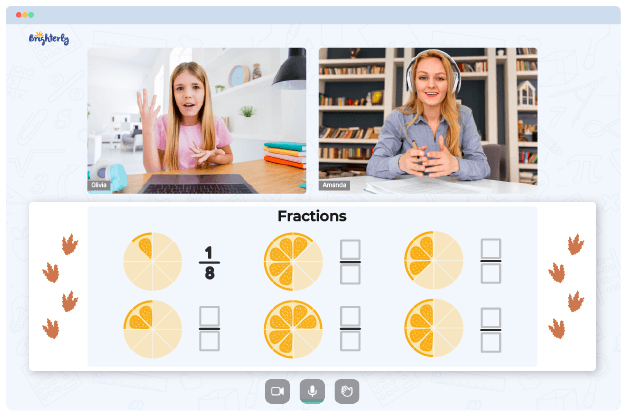# Multiplying Mixed Numbers – Definition With Examples

At Brighterly, we believe that mastering the skill of multiplying mixed numbers can open up a whole new world of problem-solving and real-life applications. Learning to multiply mixed numbers might appear challenging initially, but with a little perseverance and our step-by-step guidance, it becomes an enjoyable and effortless math skill for children to acquire. In this article, we will deconstruct the process of multiplying mixed numbers, making it simpler and more accessible for young learners. So, let’s embark on this exciting journey through the realm of mixed numbers and uncover the various techniques of multiplication.

## What Are Mixed Numbers

Mixed numbers are numbers that have both a whole number part and a fractional part. They are commonly used to represent quantities that are not whole numbers, such as lengths, weights, or volumes. For example, 2 1/2 (two and one-half) is a mixed number because it contains a whole number (2) and a fraction (1/2).

Mixed numbers are a useful way to represent and compare quantities that are not exactly whole numbers. They help us understand the world around us better and make it easier to solve real-life problems involving fractions.

## How to Multiply Mixed Numbers?

When it comes to multiplying mixed numbers, there are a few simple steps to follow:

1. Convert the mixed numbers to improper fractions.
2. Multiply the improper fractions.
3. Simplify the result, if necessary.
4. Convert the result back to a mixed number, if desired.

In the following sections, we will explore each of these steps in more detail and provide examples to help solidify your understanding.### Mixed Number To Improper Fraction Worksheet PDF

Mixed Number To Improper Fraction Worksheet### Mixed Numbers To Improper Fractions Worksheet PDF

Mixed Numbers To Improper Fractions Worksheet

To strengthen your comprehension of the concept of Mixed Numbers, we recommend checking out the collection of math worksheets available at Brighterly. These worksheets are intended to support your learning and practice of this topic.

## Multiplying Two or More Mixed Numbers

To multiply two or more mixed numbers, first convert them to improper fractions. An improper fraction is a fraction where the numerator (top number) is greater than or equal to the denominator (bottom number). To convert a mixed number to an improper fraction, use the following formula:

`Improper Fraction = (Whole Number × Denominator) + Numerator / Denominator`

Once you have converted the mixed numbers to improper fractions, simply multiply the numerators and multiply the denominators. Finally, simplify the resulting fraction, if possible.

## Multiplying a Mixed Number with Fraction

When multiplying a mixed number with a fraction, the process is very similar to multiplying two mixed numbers. First, convert the mixed number to an improper fraction. Then, multiply the improper fraction and the given fraction. As always, simplify the result if necessary.

## Multiplying a Mixed Number by a Whole Number

Multiplying a mixed number by a whole number is a straightforward process. First, convert the mixed number to an improper fraction. Next, multiply the numerator of the improper fraction by the whole number, keeping the denominator unchanged. Finally, simplify the result and convert it back to a mixed number, if desired.

## Practice Questions on Multiplying Fractions with Mixed Numbers

1. Multiply 2 1/2 by 3 3/4.
2. Multiply 4 1/3 by 1/2.
3. Multiply 5 by 1 2/3.

## Conclusion

The journey to mastering mixed numbers multiplication may seem daunting at first, but with the right mindset and consistent practice, it becomes an indispensable and manageable math skill. By following the straightforward steps provided in this Brighterly article, you can effortlessly multiply mixed numbers and apply this valuable knowledge to a myriad of real-life situations.

At Brighterly, our goal is to empower children to tackle complex mathematical concepts with confidence and enthusiasm. As they learn to multiply mixed numbers, they will not only develop a solid foundation in mathematics but also gain the ability to think critically and creatively when faced with real-world challenges. So, let’s continue learning and growing together on this exciting mathematical adventure!

## Frequently Asked Questions on Multiplying Fractions with Mixed Numbers

### How do I multiply mixed numbers with different denominators?

• Convert the mixed numbers to improper fractions, find a common denominator, and then multiply the numerators and denominators.

### Can I multiply mixed numbers without converting them to improper fractions?

• While it’s possible to multiply mixed numbers using the distributive property, converting them to improper fractions simplifies the process and is the recommended method.

### How do I know if my result is in simplest form?

• After multiplying the fractions, check if the numerator and denominator have any common factors. If they do, divide both by the greatest common factor to simplify the fraction.

### What if my result is an improper fraction? Should I convert it back to a mixed number?

• Yes, it’s usually a good idea to convert the result back to a mixed number, especially if the context of the problem involves mixed numbers or whole numbers.

### Can I use the same process for dividing mixed numbers?

• Yes, but you will need to multiply by the reciprocal of the second mixed number (or fraction) instead of directly multiplying. The overall process remains similar.
Information Sources

Remember, practice makes perfect. By regularly working on problems involving multiplying mixed numbers, children will become more comfortable with this concept and gain confidence in their math skills. Happy learning!

Problems with Fractions?• Does your child need extra support with understanding fractions fundamentals?
• Start lessons with an online tutor.

Is your child finding it hard to master the concept of fractions? An online tutor could be of help.